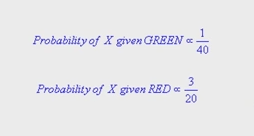# Naive Bayes Classifier

Last updated on Nov 30,2020 4K Views## Naive Bayes Classifier

Based on the Bayesian theorem, Naive Bayes Classifier is a simple probabilistic classifier with strong independent assumptions. It assumes that the presence or absence of a particular feature is unrelated to the presence or absence of another feature. A fruit may be considered to be an apple if it is red in colour, round and about 4 in diameter. Even if these features depend upon the existence of another feature, the classifier considers all of these properties to contribute to the fruit being an apple.

## Example

Above is an example mention and the objects are classified as green and red. The task is to classify new cases that arrive and understand to which class they will belong i.e. red or green.

Here we see that there are twice as many green objects as red and it is reasonable to believe that the new object is likely to be associated with green rather than red. In the bayes theory, it is known as prior probability. Prior probabilities are based on previous experiences and are used to predict outcomes before they actually happen. Thus we can write

Prior probability of green= Number of green objects     = 40

Total number of objects          60

Prior probability of red= Number of red objects = 20

Total number of objects  60

Determine Prior ProbabilityWe are now ready to classify the prior probability. As you can see in the diagram there is a white object (X) and a circle drawn around it. The circle is called the vicinity of X. As discussed earlier, the new object i.e. the white object is likely to belong to green since it is high in number, but in the vicinity of X, the number of reds in the vicinity is more as compared to green. From this we calculate the likelihood:The likelihood further indicates that the class membership of X is red since there are more red in the vicinity of X. According to Bayes classification, the final outcome is judged by combining the prior and the likelihood to achieve a Posterior Probability.Finally, we classify X to belong to red since its posterior probability is high.

Got a question for us? Mention them in the comments section and we will get back to you.

Related Posts:

Association Rule mining with Data Science

Applying Hadoop with Data Science

Get Started with Data Science

Comments
1 CommentREGISTER FOR FREE WEBINARThank you for registering Join Edureka Meetup community for 100+ Free Webinars each month